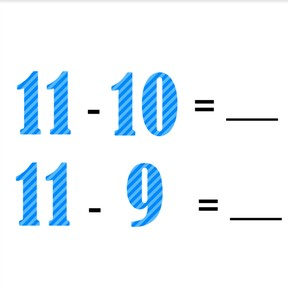Subtraction to 20 with one more or one less, decomposing the second number

# Subtraction to 20 with one more or one less, decomposing the second number8,000 schools use Gynzy92,000 teachers use Gynzy1,600,000 students use Gynzy

## General

Students learn to subtract numbers to 20 with one more or one less.

1.OA.C

## Relevance

It is useful to be able to subtract with one more or one less. If you know this you can calculate sets of subtraction problems without having to recalculate each time.

## Introduction

Give each student a number card from 1-20. Select a few students in class, and tell them that they are the starting numbers. These students must find the subsequent numbers to count to 20. Students walk through the class creating longer number lines until they reach 20. That means that there will be numerous number lines in the classroom with different starting numbers. Depending on the number of students in your classroom and starting numbers given to the lines, you can manage how many number lines will be in class. Next, count back from 20 to 10.

## Development

Show students the chocolates on the interactive whiteboard. Count the chocolates to determine that there are 18 chocolates. Show that eight of the chocolates have been eaten. That means that there are 10 chocolates left. Discuss the other images and subtraction problems shown by the chocolates. Ask students if they notice anything about the subtraction problems. The second number is one more each time. It is not always possible to have images to support math problems, sometimes you only see the numbers. You then compare the subtraction problems. If the second number in the second problem is one more than the second number in the first problem, then the difference is one less in the second problem. Show the subtraction problems on the interactive whiteboard and ask students if they can determine the answers. Also ask them the steps they took to solve the second and third subtraction problems. Did they do the calculation again, or did they just take one away from the difference? Tell students that when the second number in the second problem is is one more than the second number in the first problem, that the difference is one less. Practice subtraction problems demonstrating this.
Next, show the chocolates and determine as a class that there are 15 chocolates. If ten are eaten, that means there are 5 chocolates left. Discuss the other images and subtraction problems shown by the chocolates. Ask students if they notice anything about the subtraction problems. The second number is one less each time. It is not always possible to have images to support math problems, sometimes you only see the numbers. You then compare the subtraction problems. If the second number in the second problem is one less than the second number in the first problem then the difference is one more. Show the subtraction problems on the interactive whiteboard and ask students if they can determine the answers. Also ask them which steps they took to solve the second and third subtraction problems. Did they do the calculation again or did they just add one to the difference? Finally show subtraction problems on the interactive whiteboard and ask students to find the difference. Have them write their answers on a sheet of paper and hold them up to be checked as a class.

Check that students are able to subtract numbers to 20 with one more or one less by asking the following questions:
- What must you look at to see if you need to add one more or take one away?
- Calculate this set: 15 - 3 =, 15 - 4 =, and 15 - 5 =
- Calculate this set: 16 - 6 =, 16 - 5, and 16 - 4 =

## Guided practice

Students are first asked to subtract with one more or one less with a set of two subtraction problems. They are then given sets of three and four subtraction problems to solve using one more or one less.

## Closing

Discuss with students that it is important to be able to subtract numbers to 20 with one more or one less so you can easily solve sets of calculations. Show the subtraction problem on the interactive whiteboard and ask students to determine what the subtraction problems with one more are. Then they must determine the subtraction problems with one less for another subtraction problem. Finally students are given a selection of subtraction problems. They must be dragged to match in order of one more or one less and then solved as a class.

## Teaching tips

Students who have difficulty with subtracting with one more or one less can make use of manipulatives like MAB blocks. Have them set out the total number of blocks and then remove the given number. Then have them practice with making that number one more or one less.

## Instruction materials

Number cards to 20
optional: manipulatives like MAB blocks

### The online teaching platform for interactive whiteboards and displays in schools

• Save time building lessons

• Manage the classroom more efficiently

• Increase student engagement